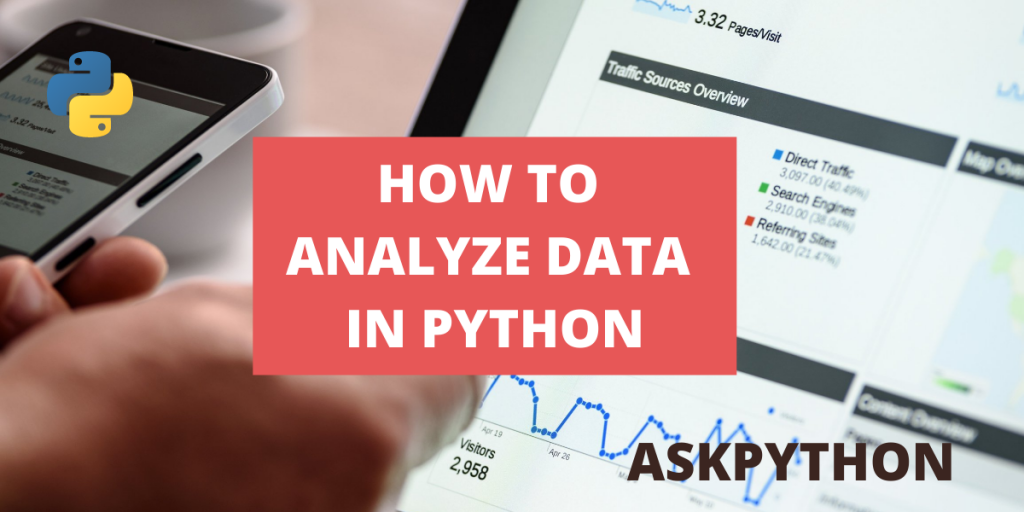# Data Analysis in Python – A Quick IntroductionLet’s learn more about Data Analysis in Python. Analyzing data is quite easy if you have a grasp of Python. There are a lot of packages available for the same.

In this article, we will be looking at the different python packages, tools, methods that aid us in data analysis. We will start by looking at how different forms of datafiles- from excel sheets to online databases can be imported into python source code and then we will look at the method through which those data can be implemented into different kinds of graphs.

## Working On Data Analysis in Python

Before we read any data, first we need to grasp the know-how of how to load different types of files in python, and then we can proceed ahead.

### Load Local Data Sets In Python

In this example, the program loads the .csv file from the same directory from where the python script is run.

```import pandas as pd
```

### Load Datasets From URL In Python

As it is clear from the code below, the ‘pd.read’ syntax easily loads the .csv file through the given URL.

```import pandas as pd
print(df)
```

### Load Excel Data In Python

The ‘pd.read_excel’ syntax is similar to the previously used ‘pd.read’ syntax, and it is used to load excel sheets into our python program. We used an excel sheet (‘data.xlsx’ present in the same directory through which the python code is run, and we used ‘openpyxl’ engine, which is an excel interpreter for python.

```import pandas as pd
```

## How to Analyze Data in Python Using Different Charts?

Since the time the concept of numbers was created, humans have created multiple methods to make counting numbers easier. But never made understanding numbers easier than graphs and charts did. In data analysis too, we will be looking at python modules that help in creating graphs and diagrams using the datafiles we loaded.

### 1. Pie Charts

Pie Charts are 360-degree graphical representations of two different sets of data, shown together to display a confluence. In the code below, the program will plot a piechart with two sets of values – ‘Age’, ‘Pregnancies’.

Code:

```# import statements
import plotly.express as fpx
import pandas as pd

preg_stats = fdf['Pregnancies']
age_stats = fdf['Age']

fig = fpx.pie(fdf,
values=preg_stats,
names=age_stats,
title='Survey Results'
)

fig.update_traces(
textposition='inside',
textinfo='percent+label'
)

fig.update_layout(
title_font_size = 42,
)

fig.show()
```

### 2. Line Charts

The line chart is drawn to understand the relation between two sets of values. In the code below, the program plots the line chart and displays output.

Code:

```# import statements
import matplotlib.pyplot as plt
import pandas as pd

#Sorting the two columns in ascending order
fdf.sort_values(["Age", "Pregnancies"],
axis=0,
inplace=True)

preg_stats = fdf['Pregnancies']
age_stats = fdf['Age']

plt.plot(age_stats,preg_stats)
plt.show()
```

Also read: Plot data from Excel Sheet using Python

### 3. Scatter

The Scatter function in matplotlib distributes values in a 2-D plane and visualizes a graphical representation of it. This representation is suitable to analyze properties like density, random distribution for a set of values. Representation can also be made for more than one variable.

In this example, a consumer record database is used to compile the results and produce a scatter graph. The code below visualizes a scatter graph for a set of two variables from that database.

Code:

```import pandas as pd
import matplotlib.pyplot as plt

plt.style.use('seaborn')

cid = data['Clothing ID']
age = data['Age']
rating = data['Rating']

plt.scatter(age, rating, cmap='summer',
edgecolor='black', linewidth=1, alpha=0.75)

cbar = plt.colorbar()
cbar.set_label('Age/Rating Ratio')

plt.xscale('log')
plt.yscale('log')

plt.title('Age vs Rating')
plt.xlabel('Age ->')
plt.ylabel('Rating ->')

plt.tight_layout()

plt.show()
```

### 4. Histogram

The histogram is a graphical representation of the distribution of frequency and it is displayed with adjoining bars. The histogram function The histogram is a graphical picture of the dispersal of frequency and it is exhibited with adjoining bars. The histogram function of matplotlib plots the frequency points from a single variable and demonstrates them in the output.

Code:

```import matplotlib.pyplot as plt
import pandas as pd

age = data['Age']

plt.hist(age)
plt.show()
```

### 5. Bar Graph

Rectangular representation of two sets of variables represented both horizontally and vertically.

Code:

```import matplotlib.pyplot as plt
import pandas as pd

cid = data['Clothing ID']
age = data['Age']

plt.barh(age, cid)
plt.show()```